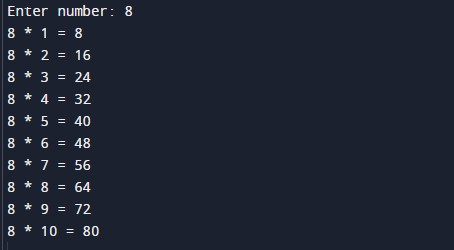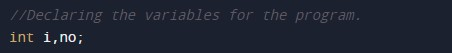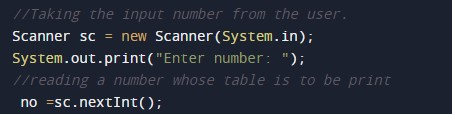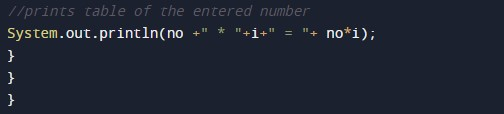# Java Program to Print Table of any Number

In this tutorial you will learn about the Java Program to Print Table of any Number and its application with practical example.

## Java Program to Print Table of any Number

In this tutorial, we will learn to create a Java program that will print a Table of any Number using Java programming.

## Prerequisites

Before starting with this tutorial, we assume that you are the best aware of the following Java programming topics:

• Operators in Java Programming.
• Basic Input and Output function in Java Programming.
• Basic Java programming.
• Conditional Statements in Java programming.
• Arithmetic operations in Java Programming.

## Program to Print Table of any number:-

In today’s program, we will take input in number format. Secondly, we will print a Table of any number with the help of a small program. The numbers are the most useful terminology in any programming language. For number/arithmetic manipulation in Java programming, there are many predefined functions available.

With the help of this program, we can take input and print the Table of any Number.

## Output:-In the above program, we have first initialized the required variable.• no = it will hold the input number value from the user.
• i  = it will hold the integer value.

Taking input numbers from the user.Calculating the table of given numbers using a for loop.In this tutorial we have learn about the Java Program to Print Table of any Number and its application with practical example. I hope you will like this tutorial.